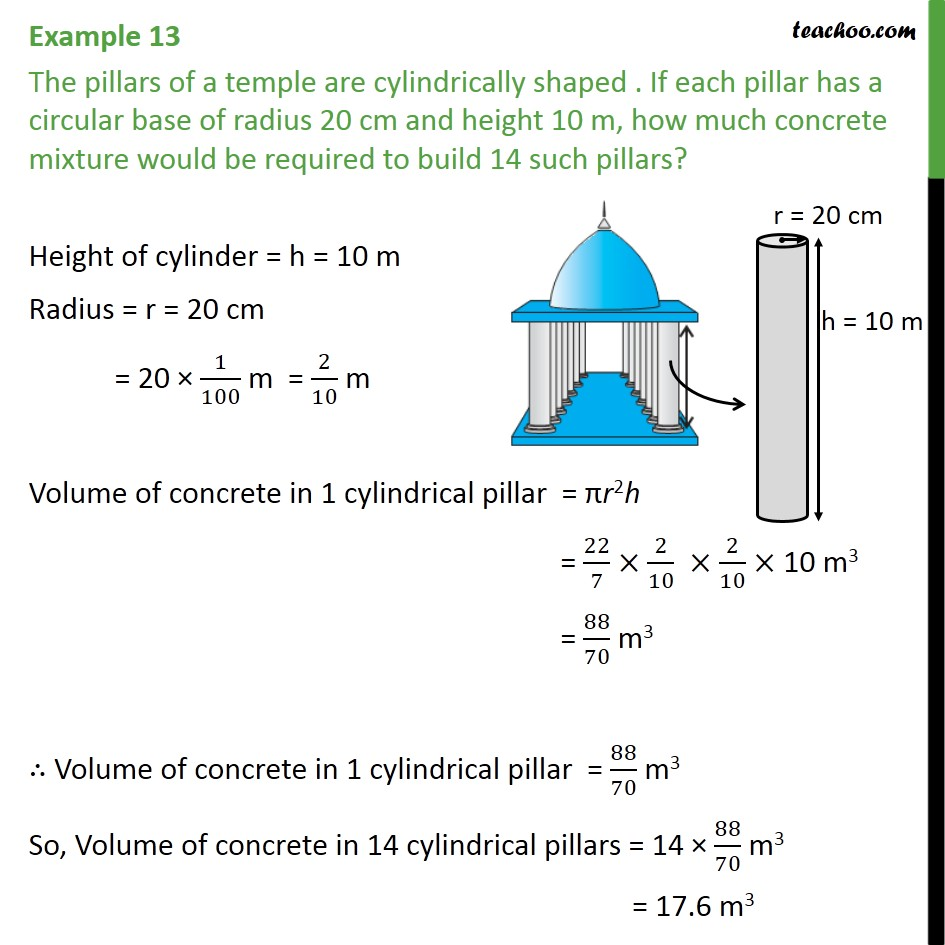1. Chapter 13 Class 9 Surface Areas and Volumes (Term 2)
2. Serial order wise
3. Examples

Transcript

Example 13 The pillars of a temple are cylindrically shaped . If each pillar has a circular base of radius 20 cm and height 10 m, how much concrete mixture would be required to build 14 such pillars? Height of cylinder = h = 10 m Radius = r = 20 cm = 20 × 1/100 m = 2/10 m Volume of concrete in 1 cylindrical pillar = πr2 h = 22/7 × 2/10 × 2/10 × 10 m3 = 88/70 m3 ∴ Volume of concrete in 1 cylindrical pillar = 88/70 m3 So, Volume of concrete in 14 cylindrical pillars = 14 × 88/70 m3 = 17.6 m3